1260

Hello eveyone!

I was wondering on how a NN works in forex and how to "train" it depending on the peformance.

The idea is this: based on 3 indicators, I create a NN that consist on 4 neurons(N).

N1 recieves data from indicator 1 and 2.

N2 recieves data from indicator 1 and 3.

N3 recieves data from indicator 2 and 3

N4 recieves data from indicator 1, 2 and 3.

Then, the result of each neuron gets multiplied by other weights and then goes to the output.

All weights are started as random numbers when the EA starts.

The next step, is to apply a sigmoid function to the values and, if the value is greater than a bias, activate a buy or sell operation, depending on the current state.

If the operation results in a loss, it is obviously desired to apply a correction to the weights, since we can't modify the inputs.

And here my question. How does the backpropagation function works? As far as I have read, its a function that corrects the weights based on the costs of the function (sigmoid function I think).

The main idea is: when the result is negative, use backpropagation for adjusting the weights and when the result is positive use the same idea to give a reward to the NN.

Any ideas? Thoughts?

I'm posting how I created the neuron and the sigmoid function I'm using. Is this something that has the potential of giving good results?

```double activateNN()
{
double ind_1=/*insert indicator*/;
double ind_2=/*insert indicator*/;
double ind_3=/*insert indicator*/;

//NN1
double NN1=0,NN2=0,NN3=0,NN4=0;

NN1   =  ind_1 *  weight   +  ind_2 *  weight;
NN2   =  ind_1 *  weight   +  ind_3 *  weight;
NN3   =  ind_2 *  weight   +  ind_3 *  weight;
NN4   =  ind_1 *  weight   +  ind_2 *  weight   +  ind_3 *  weight;

outputs   =  Sigmoid1(NN1);
outputs   =  Sigmoid1(NN2);
outputs   =  Sigmoid1(NN3);
outputs   =  Sigmoid1(NN4);

double out1=0,out2=0,out3=0,out4=0;

out1  =  outputs   *  weight;
out2  =  outputs   *  weight;
out3  =  outputs   *  weight;
out4  =  outputs   *  weight;

double totSum=0;

totSum=out1+out2+out3+out4;
outputs=Sigmoid1(totSum);

}```
```double Sigmoid1(double A)
{
return (2*A)/(2 + MathAbs(A));
}```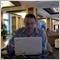Moderator
118951

### Neural Network

1. Better NN EA development thread with indicators, pdf files and so on.
2. Better NN EA final thread
3. Neural Networks thread (good public discussion)
4. How to build a NN-EA in MT4: usefull thread for developers.
5. Radial Basis Network (RBN) - As Fit Filter For Price: the thread

Neural Network: Indicators and systems development

1. Self-trained MA cross!: development thread for new generation of the indicators

Neural Network: EAs

2. Self learning expert thread with EAs' files here.
3. Artificial Intelligence EAs threads: How to "teach" and to use the AI ("neuron") EA thread and Artificial Intelligence  thread
4. Forex_NN_Expert EA and indicator thread.
5. SpiNNaker - A Neural Network EA thread

Neural Network: The Books

1. What to read and where to learn about Machine Learning (10 free books) - the post.

The article

CodeBase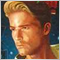740

Pedro Severin:

And here my question. How does the backpropagation function works? As far as I have read, its a function that corrects the weights based on the costs of the function (sigmoid function I think).

The main idea is: when the result is negative, use backpropagation for adjusting the weights and when the result is positive use the same idea to give a reward to the NN.

Any ideas? Thoughts?

Sounds to me your aim is to self-optimize.

My advice is experiment with the NN first. You can train the network with the optimizer. The good thing with NN's is that is very efficient in coming up with good answers, this is also the pitfall. Unless you know what you are doing, NN are very prone to curve fitting (over fitting). Most if not all examples posted by Sergey fit the category garbage in garbage out. You need to ask the right question in order to get the right answer.

Experiment first, then complicate things with self optimizing because it is a whole subject of its own. For example you need large enough sample sizes. Self optimizing on a trade by trade bases is too slow, you would need some type of virtual trading environment to speed up the self optimizing process. Take into consideration the amounts of weights times the weight bandwidth the combinations add up real fast and thus can become rather resource intensive.11935

Pedro Severin:

Hello eveyone!

I was wondering on how a NN works in forex and how to "train" it depending on the peformance.

The idea is this: based on 3 indicators, I create a NN that consist on 4 neurons(N).

N1 recieves data from indicator 1 and 2.

N2 recieves data from indicator 1 and 3.

N3 recieves data from indicator 2 and 3

N4 recieves data from indicator 1, 2 and 3.

Then, the result of each neuron gets multiplied by other weights and then goes to the output.

All weights are started as random numbers when the EA starts.

The next step, is to apply a sigmoid function to the values and, if the value is greater than a bias, activate a buy or sell operation, depending on the current state.

If the operation results in a loss, it is obviously desired to apply a correction to the weights, since we can't modify the inputs.

And here my question. How does the backpropagation function works? As far as I have read, its a function that corrects the weights based on the costs of the function (sigmoid function I think).

The main idea is: when the result is negative, use backpropagation for adjusting the weights and when the result is positive use the same idea to give a reward to the NN.

Any ideas? Thoughts?

I'm posting how I created the neuron and the sigmoid function I'm using. Is this something that has the potential of giving good results?

Backprop works the opposite direction. From a known output (constant) & its indicators values (constant) at instant T you deduce weights that suits the ouput (variable).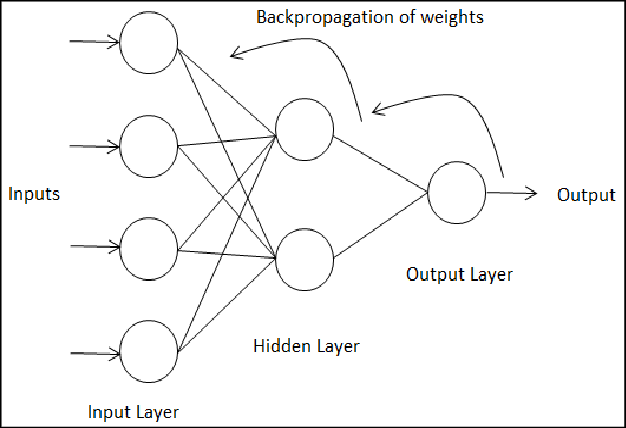It seems easy said so but it's f*king complicated to code entirely considering all layers, I strongly suggest you to use the new python integration for it :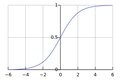A Neural Network in 11 lines of Python (Part 1) - i am trask
Summary: I learn best with toy code that I can play with. This tutorial teaches backpropagation via a very simple toy example, a short python implementation. Edit: Some folks have asked about a followup article, and I'm planning to write one. I'll tweet it out when it's complete at @iamtrask. Feel free to follow if you'd be interested in...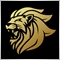Moderator
13053

I would never use indicator data for NN.

Just the raw price data only.1260

Marco vd Heijden:

I would never use indicator data for NN.

Just the raw price data only.

Hi,
Why only raw prices?1260

Enrique Dangeroux:

Sounds to me your aim is to self-optimize.

My advice is experiment with the NN first. You can train the network with the optimizer. The good thing with NN's is that is very efficient in coming up with good answers, this is also the pitfall. Unless you know what you are doing, NN are very prone to curve fitting (over fitting). Most if not all examples posted by Sergey fit the category garbage in garbage out. You need to ask the right question in order to get the right answer.

Experiment first, then complicate things with self optimizing because it is a whole subject of its own. For example you need large enough sample sizes. Self optimizing on a trade by trade bases is too slow, you would need some type of virtual trading environment to speed up the self optimizing process. Take into consideration the amounts of weights times the weight bandwidth the combinations add up real fast and thus can become rather resource intensive.

Indeed, I want to avoid curve fitting. I don't know if self optimizing is what it should be doing, maybe more like learning by auto adjusting the weights.

Why do you say that cyberia trader does not work? Is a bad ea? Or anyone that Sergey posted?740

Pedro Severin:
Indeed, I want to avoid curve fitting. I don't know if self optimizing is what it should be doing, maybe more like learning by auto adjusting the weights.

Why do you say that cyberia trader does not work? Is a bad ea? Or anyone that Sergey posted?

Most of them are based on indicator connected with NN, this is futile because instead of optimizing parameters you would be optimizing the network either backwards or forwards, does not matter. No difference there. Besides Indicators are a bad idea because all of them are not profitable to begin with from a long term statistical perspective. I too would never use an indicator for NN input.

Raw prices is better but prices only from asset you are trading does not make any sense either. Better try to design a structure to incorporate more factors which impact price. Such as OIL for trading CAD or AUD, correlation between assets, or a triangle of assets Such as EURUSD/GBPUSD/EURGBP. Problem with that is that in can become complicated very fast, and the risk of over fitting is still there if you do not know what you are doing.

Toy with some of the EA's posted by Sergey (examine code carefully, many make logic mistakes, especially code posted in codebase) see if you can improve them, or make very simple test to see how things work like trying to forecast next day direction based on D1 OHLC data. It is not easy to make it perform better then a coin flip but you will learn a lot.1260

Enrique Dangeroux:

Most of them are based on indicator connected with NN, this is futile because instead of optimizing parameters, you would be optimizing the network either backwards of forwards, does not matter, no difference there. Besides Indicators are a bad idea because all of them are not profitable to begin with from a long term statistical perspective. I too would never use an indicator for NN input.

Raw prices is better but prices only from asset you are trading does not make any sense either. Better try to design a structure to incorporate more factors which impact price. Such as OIL for trading CAD or AUD, correlation between assets, or a triangle of assets Such as EURUSD/GBPUSD/EURGBP. Problem with that is that in can become complicated very fast, and the risk of over fitting is still there if you do not know what you are doing.

Toy with some of the EA's posted by Sergey (examine code carefully, many make logic mistakes, especially code posted in codebase) see if you can improve them, or make very simple test to see how things work like trying to forecast next day direction based on OHLC data. It is not easy to make it perform better then a coin flip but you will learn a lot.

I see. Thank you for such nice feedback. Do you think every indicator is going to be a failure? For example, how about the ATR? Personally, I found this indicator very different from others, because it doesn't shows the direction, only how much price is moving.

On the other side, isn't that a good thing that the EA is optimizing constantly, even if it's based on indicators? I know that curve fitting is a problem, but how about if you have an EA that you optimize it every week and change setting based on that every week? Wouldn't you be getting the best results in the near future?

I always liked the optimization concept, but what I think is that you will get the best parameters for that period of time, and I think they might work for a short period forward. How short? I don't know. Might be a week, month or even a year. So, when optimizing, what should I be looking for and how should I do a correct optimization (and how often should I do it)Moderator
13053

Pedro Severin:
Hi,
Why only raw prices?

It's the obvious choice.

You want it to train properly without the use of ANY indicators.1260

Marco vd Heijden:

It's the obvious choice.

You want it to train properly without the use of ANY indicators.

I don't get it...I mean, for example, moving averages are based on prices (close price, open price, high,low,typical,etc). ATR is based on price also, many indicators are price based, so why an indicator like this would be a bad choice?

And, for example, if only raw prices, you would take prices of the last candles? The highs,lows,closes? Or tick prices that are coming right now? And how would you use the backpropagation function in this case?